Courses

# Test: Sigmatropic

## 30 Questions MCQ Test Physical Chemistry | Test: Sigmatropic

Description
This mock test of Test: Sigmatropic for Chemistry helps you for every Chemistry entrance exam. This contains 30 Multiple Choice Questions for Chemistry Test: Sigmatropic (mcq) to study with solutions a complete question bank. The solved questions answers in this Test: Sigmatropic quiz give you a good mix of easy questions and tough questions. Chemistry students definitely take this Test: Sigmatropic exercise for a better result in the exam. You can find other Test: Sigmatropic extra questions, long questions & short questions for Chemistry on EduRev as well by searching above.
QUESTION: 1

Solution:
QUESTION: 2

Solution:
QUESTION: 3

### The major product formed in the thermal reaction given below, is: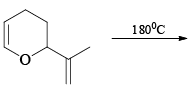Solution:
QUESTION: 4

The major product P of the given reaction is: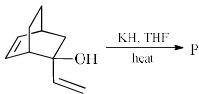Solution:
QUESTION: 5

In the reaction the major products X and Y are: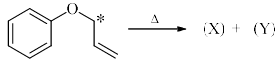Solution:
QUESTION: 6

In the reaction, the major product (X) is: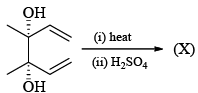Solution:
QUESTION: 7

The pericyclic reaction given below is an example of: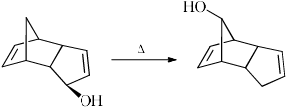Solution:
QUESTION: 8

Claisen rearrangement is an example of:

Solution:
QUESTION: 9

The product of the following reaction is: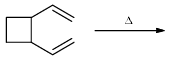Solution:
QUESTION: 10

In sigmatropic thermal expansion of 1, 1-dicyclopropyl ethene, the final product:

Solution:
QUESTION: 11

Give the structure of the product in the following reaction: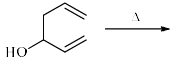Solution:
QUESTION: 12

This sigmatropic reaction is example of: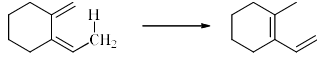Solution:
QUESTION: 13

The following transformation proceeds by the sequence indicated. Give the structure of product: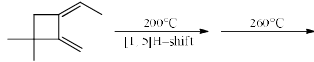Solution: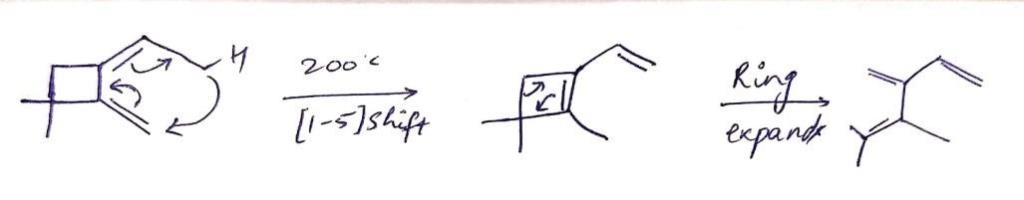Hence C is the correct answer.

QUESTION: 14

Allyl aryl ether on heating at 200°C give the major product:

Solution:
QUESTION: 15

Which of adducts (a)-(d) is the main product of the following Diels-Alder reaction?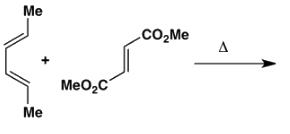Solution:
QUESTION: 16

Consider the following statements:

(I) Clasisen rearrangement is a [3, 3] sigmatropic rearrangement.
(II) Cope rearrangement proceeds via is chair like transition state.
(III) In the photochemical [2 + 2] cycloaddition of ethylene overlapping of HOMO of one molecule with LUMO of the other molecular takes place.

Select the correct statements.

Solution:
QUESTION: 17

The product is: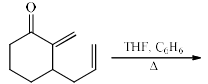Solution:
QUESTION: 18

The major product of the following reaction is: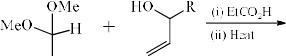Solution:
QUESTION: 19

The major product of the following reaction is: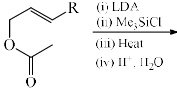Solution:
QUESTION: 20

In which of the following reaction, which sigmatropic reaction will take place: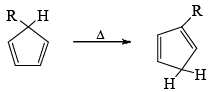Solution:
QUESTION: 21

The reaction given below is an example of: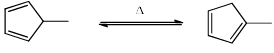Solution:
QUESTION: 22

Among the following dienes, the one that undergoes a degenerate Cope rearrangement is:

Solution:
QUESTION: 23

Pericyclic reaction involved in one of the steps of the following reaction sequence is: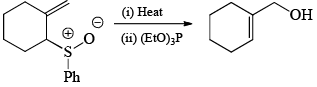Solution:
QUESTION: 24

The product formed and the process involved in the following reaction is: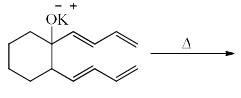Solution:
QUESTION: 25

The two step conversion of 7-dehydrocholesterol to vitamin D3 proceeds through: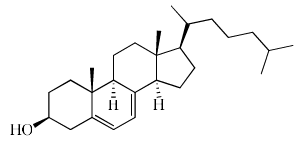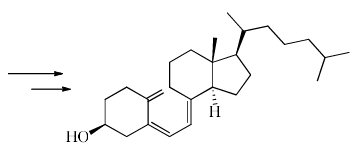Solution:
QUESTION: 26

The major product formed in the following reaction is: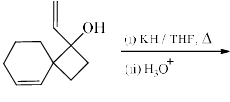Solution:
QUESTION: 27

With respect to the following biogenetic conversion of chorismic acid (A) to 4-ydroxyphenylpyruvic acid (C), the correct statement is: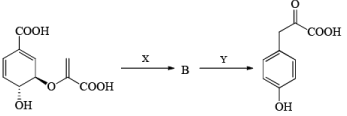Solution:
QUESTION: 28

Formation of the ketone (II) fro m the diazoketone (I) involves: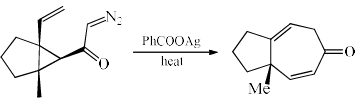Solution:
QUESTION: 29

The reaction is given below is an example of: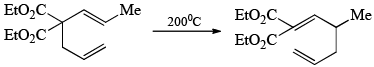Solution:
QUESTION: 30

The major product is: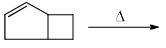Solution: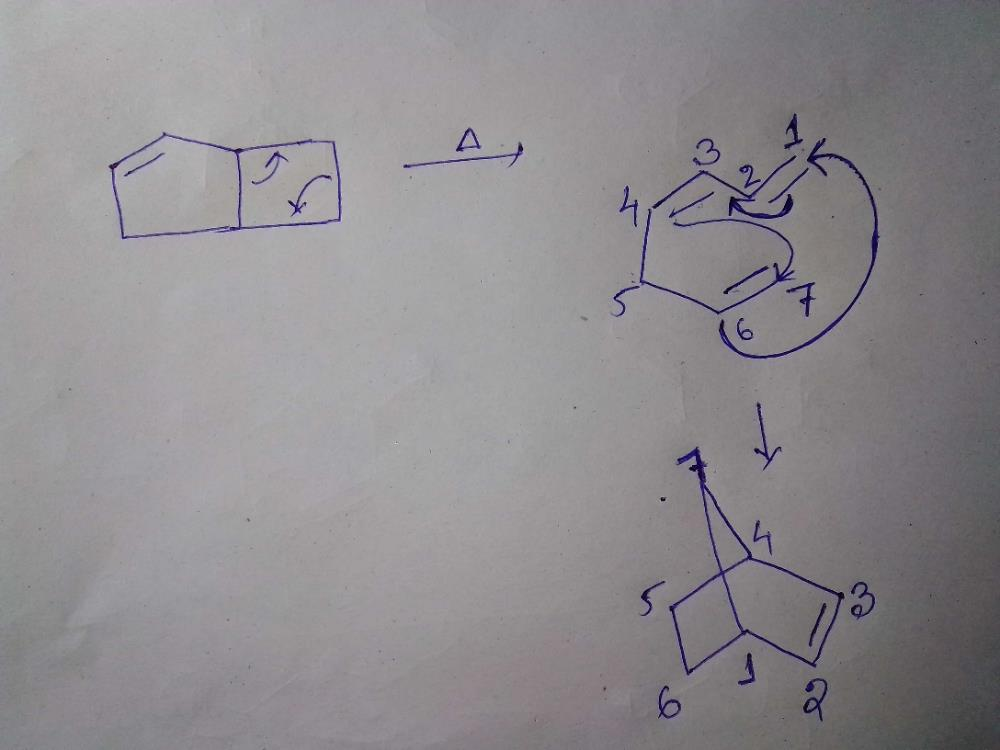• Test